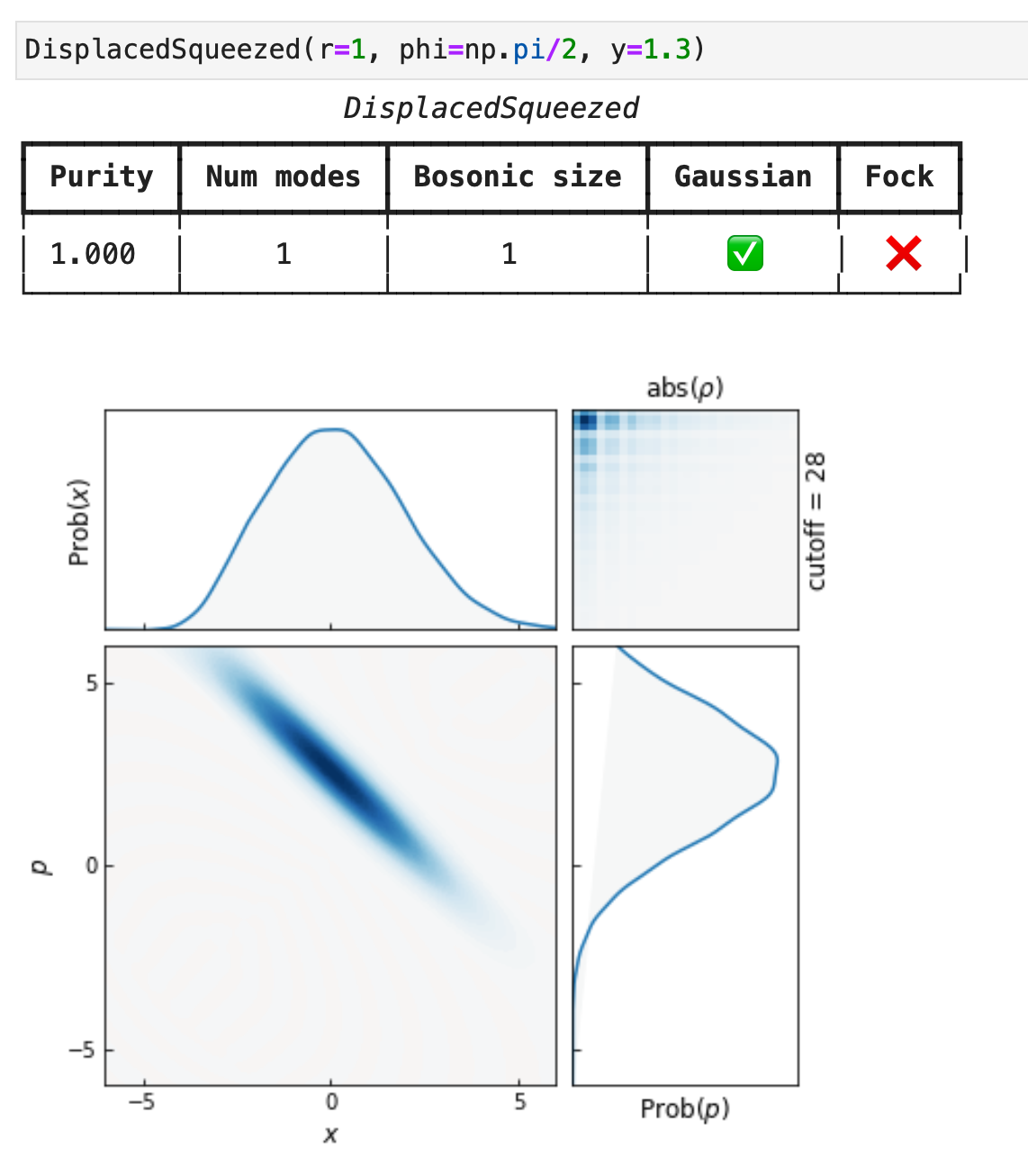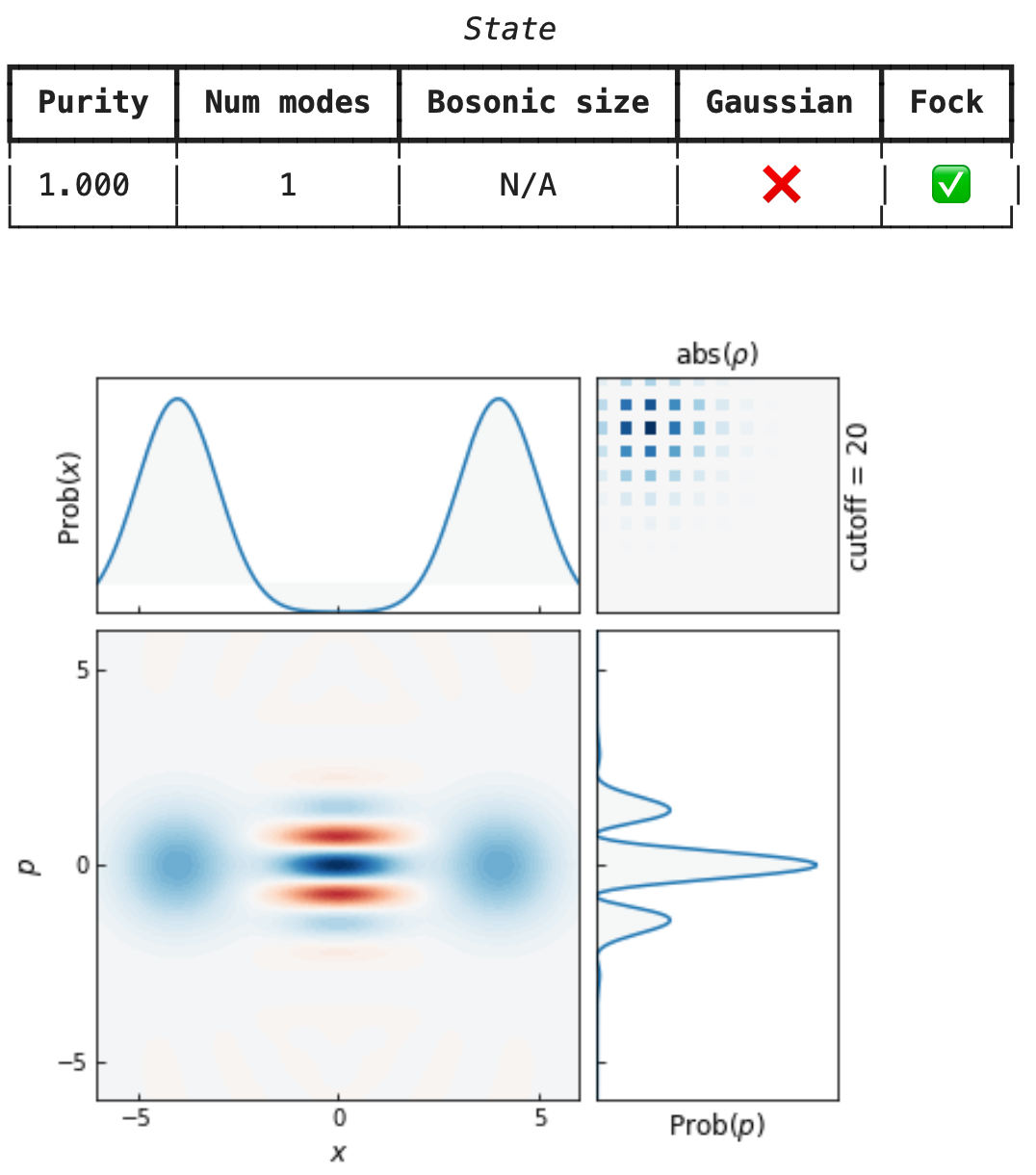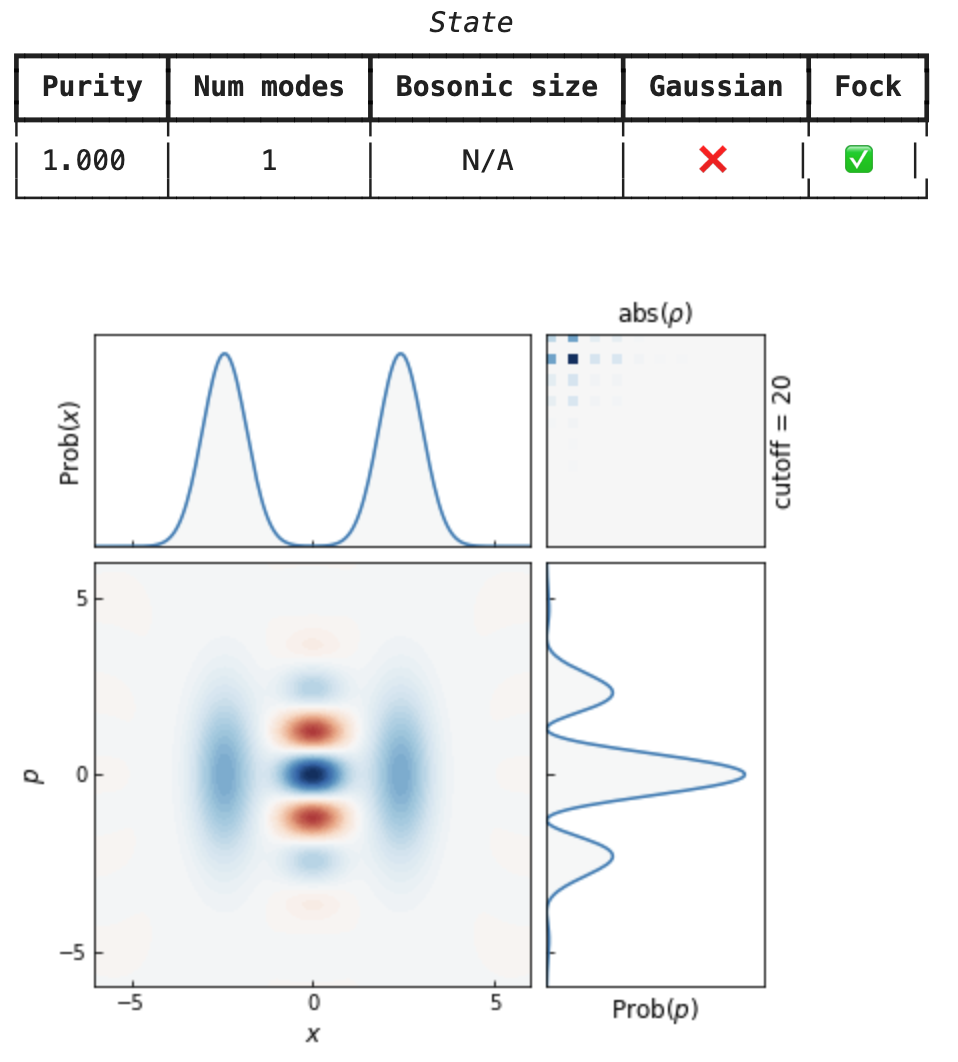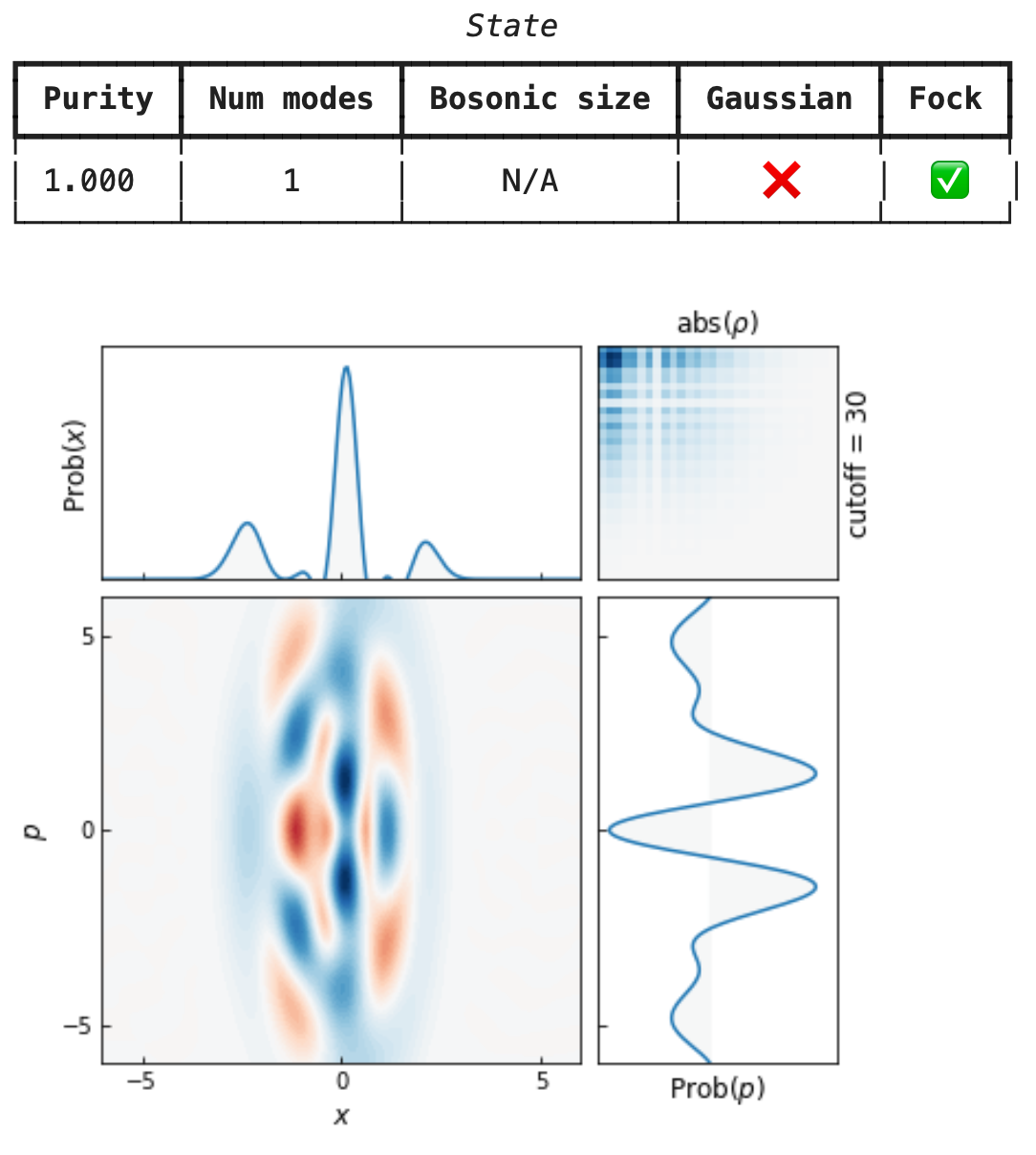# Basic API Reference¶

## 1. States and Gates¶

Here are a few examples of states and gates:

import numpy as np
from mrmustard.lab import *

vac = Vacuum(num_modes=2)        # 2-mode vacuum state
coh = Coherent(x=0.1, y=-0.4)    # coh state |alpha> with alpha = 0.1 - 0.4j
sq  = SqueezedVacuum(r=0.5)      # squeezed vacuum state
g   = Gaussian(num_modes=2)      # 2-mode Gaussian state with zero means
fock4 = Fock(4)                  # fock state |4>

D  = Dgate(x=1.0, y=-0.4)         # Displacement by 1.0 along x and -0.4 along y
S  = Sgate(r=0.5)                 # Squeezer with r=0.5

BS = BSgate(theta=np.pi/4)          # 50/50 beam splitter
S2 = S2gate(r=0.5)                  # two-mode squeezer
MZ = MZgate(phi_a=0.3, phi_b=0.1)   # Mach-Zehnder interferometer
I  = Interferometer(8)              # 8-mode interferometer
L  = Attenuator(0.5)                # pure lossy channel with 50% transmissivity
A  = Amplifier(gain=2.0, nbar=1.0)  # noisy amplifier with 200% gain


The repr of single-mode states shows the Wigner function:cat_amps = Coherent(2.0).ket() + Coherent(-2.0).ket()
cat_amps = cat_amps / np.linalg.norm(cat_amps)
cat = State(ket=cat_amps)
catStates (even those in Fock representation) are always compatible with gates:

cat >> Sgate(0.5)  # squeezed cat## 2. Gates and the right shift operator >>¶

Applying gates to states looks natural, thanks to python’s right-shift operator >>:

displaced_squeezed = Vacuum(1) >> Sgate(r=0.5) >> Dgate(x=1.0)


If you want to apply a gate to specific modes, use the getitem format. Here are a few examples:

D = Dgate(y=-0.4)
S = Sgate(r=0.1, phi=0.5)
state = Vacuum(2) >> D >> S  # displacement on mode 1 and squeezing on mode 0

BS = BSgate(theta=1.1)
state = Vacuum(3) >> BS[0,2]  # applying a beamsplitter to modes 0 and 2
state = Vacuum(4) >> S[0,1,2]  # applying the same Sgate in parallel to modes 0, 1 and 2 but not to mode 3


## 3. Circuit¶

When chaining just gates with the right-shift >> operator, we create a circuit:

X8 = Sgate(r=[1.0] * 4) >> Interferometer(4)
output = Vacuum(4) >> X8

# lossy X8
noise = lambda: np.random.uniform(size=4)
X8_realistic = (Sgate(r=0.9 + 0.1*noise(), phi=0.1*noise())
>> Attenuator(0.89 + 0.01*noise())
>> Interferometer(4)
>> Attenuator(0.95 + 0.01*noise())
)

# 2-mode Bloch Messiah decomposition
bloch_messiah = Sgate(r=[0.1,0.2]) >> BSgate(-0.1, 2.1) >> Dgate(x=[0.1, -0.4])
my_state = Vacuum(2) >> bloch_messiah


## 4. Measurements¶

In order to perform a measurement, we use the left-shift operator, e.g. coh << sq (think of the left-shift on a state as “closing” the circuit).

leftover = Vacuum(4) >> X8 << SqueezedVacuum(r=10.0, phi=np.pi)  # a homodyne measurement of p=0.0 on mode 2


Transformations can also be applied in the dual sense by using the left-shift operator <<:

Attenuator(0.5) << Coherent(0.1, 0.2) == Coherent(0.1, 0.2) >> Amplifier(2.0)


This has the advantage of modelling lossy detectors without applying the loss channel to the state going into the detector, which can be overall faster e.g. if the state is kept pure by doing so.

## 5. Detectors¶

There are two types of detectors in Mr Mustard. Fock detectors (PNRDetector and ThresholdDetector) and Gaussian detectors (Homodyne, Heterodyne). However, Gaussian detectors are a thin wrapper over just Gaussian states, as Gaussian states can be used as projectors (i.e. state << DisplacedSqueezed(...) is how Homodyne performs a measurement).

The PNR and Threshold detectors return an array of unnormalized measurement results, meaning that the elements of the array are the density matrices of the leftover systems, conditioned on the outcomes:

results = Gaussian(2) << PNRDetector(efficiency = 0.9, modes = )
results  # unnormalized dm of mode 1 conditioned on measuring 0 in mode 0
results  # unnormalized dm of mode 1 conditioned on measuring 1 in mode 0
results  # unnormalized dm of mode 1 conditioned on measuring 2 in mode 0
# etc...


The trace of the leftover density matrices will yield the success probability. If multiple modes are measured then there is a corresponding number of indices:

results = Gaussian(3) << PNRDetector(efficiency = [0.9, 0.8], modes = [0,1])
results[2,3]  # unnormalized dm of mode 2 conditioned on measuring 2 in mode 0 and 3 in mode 1
# etc...


Set a lower settings.PNR_INTERNAL_CUTOFF (default 50) to speed-up computations of the PNR output.

## 6. Comparison operator ==¶

States support the comparison operator:

>>> bunched = (Coherent(1.0) & Coherent(1.0)) >> BSgate(np.pi/4)
>>> bunched.get_modes(1) == Coherent(np.sqrt(2.0))
True


As well as transformations (gates and circuits):

>>> Dgate(np.sqrt(2)) >> Attenuator(0.5) == Attenuator(0.5) >> Dgate(1.0)
True


## 7. State operations and properties¶

States can be joined using the & (and) operator:

Coherent(x=1.0, y=1.0) & Coherent(x=2.0, y=2.0)  # A separable two-mode coherent state

s = SqueezedVacuum(r=1.0)
s4 = s & s & s & s   # four squeezed states


Subsystems can be accessed via get_modes:

joint = Coherent(x=1.0, y=1.0) & Coherent(x=2.0, y=2.0)
joint.get_modes(0)  # first mode
joint.get_modes(1)  # second mode

swapped = joint.get_modes([1,0])


## 8. Fock representation¶

The Fock representation of a State is obtained via .ket(cutoffs) or .dm(cutoffs). For circuits and gates it’s .U(cutoffs) or .choi(cutoffs). The Fock representation is exact (with minor caveats) and it doesn’t break differentiability. This means that one can define cost functions on the Fock representation and backpropagate back to the phase space representation.

# Fock representation of a coherent state
Coherent(0.5).ket(cutoffs=)   # ket
Coherent(0.5).dm(cutoffs=)    # density matrix

Dgate(x=1.0).U(cutoffs=)  # truncated unitary op
Dgate(x=1.0).choi(cutoffs=)  # truncated choi op


States can be initialized in Fock representation and used as any other state:

my_amplitudes = np.array([0.5, 0.25, -0.5, 0.25, 0.25, 0.5, -0.25] + [0.0]*23)  # notice the buffer
my_state = State(ket=my_amplitudes)
my_state >> Sgate(r=0.5)  # just worksAlternatively,

my_amplitudes = np.array([0.5, 0.25, -0.5, 0.25, 0.25, 0.5, -0.25])  # no buffer
my_state = State(ket=my_amplitudes)
my_state._cutoffs =   # force the cutoff
my_state >> Sgate(r=0.5)  # works too


## The physics module¶

The physics module contains a growing number of functions that we can apply to states directly. These are made out of the functions that operate on the representation of the state:

• If the state is in Gaussian representation, then internally the physics functions utilize the physics.gaussian module.

• If the state is in Fock representation, then internally the physics functions utilize the physics.fock module.

## The math module¶

The math module is the backbone of Mr Mustard, which consists in the Math interface Mr Mustard comes with a plug-and-play backends through a math interface. You can use it as a drop-in replacement for tensorflow or pytorch and your code will be plug-and-play too!

from mrmustard import settings
from mrmustard.math import Math
math = Math()

math.cos(0.1)  # tensorflow

settings.BACKEND = 'torch'

math.cos(0.1)  # pytorch (upcoming)


## Optimization¶

The Optimizer (available in mrmustard.training uses Adam underneath the hood for Euclidean parameters and a custom symplectic optimizer for Gaussian gates and states, an unitary optimizer for interferometers, and an orthogonal optimizer for real interferometers.

We can turn any simulation in Mr Mustard into an optimization by marking which parameters we wish to be trainable. Let’s take a simple example: synthesizing a displaced squeezed state.

from mrmustard.lab import Dgate, Ggate, Attenuator, Vacuum, Coherent, DisplacedSqueezed
from mrmustard.physics import fidelity
from mrmustard.training import Optimizer

D = Dgate(x = 0.1, y = -0.5, x_trainable=True, y_trainable=True)
L = Attenuator(transmissivity=0.5)

# we write a function that takes no arguments and returns the cost
def cost_fn_eucl():
state_out = Vacuum(1) >> D >> L
return 1 - fidelity(state_out, Coherent(0.1, 0.5))

G = Ggate(num_modes=1, symplectic_trainable=True)
def cost_fn_sympl():
state_out = Vacuum(1) >> G >> D >> L
return 1 - fidelity(state_out, DisplacedSqueezed(r=0.3, phi=1.1, x=0.4, y=-0.2))

opt = Optimizer(symplectic_lr=0.1, euclidean_lr=0.01)
opt.minimize(cost_fn_eucl, by_optimizing=[D])  # using Adam for D

opt = Optimizer(symplectic_lr=0.1, euclidean_lr=0.01)
opt.minimize(cost_fn_sympl, by_optimizing=[G,D])  # using Adam for D and the symplectic opt for G